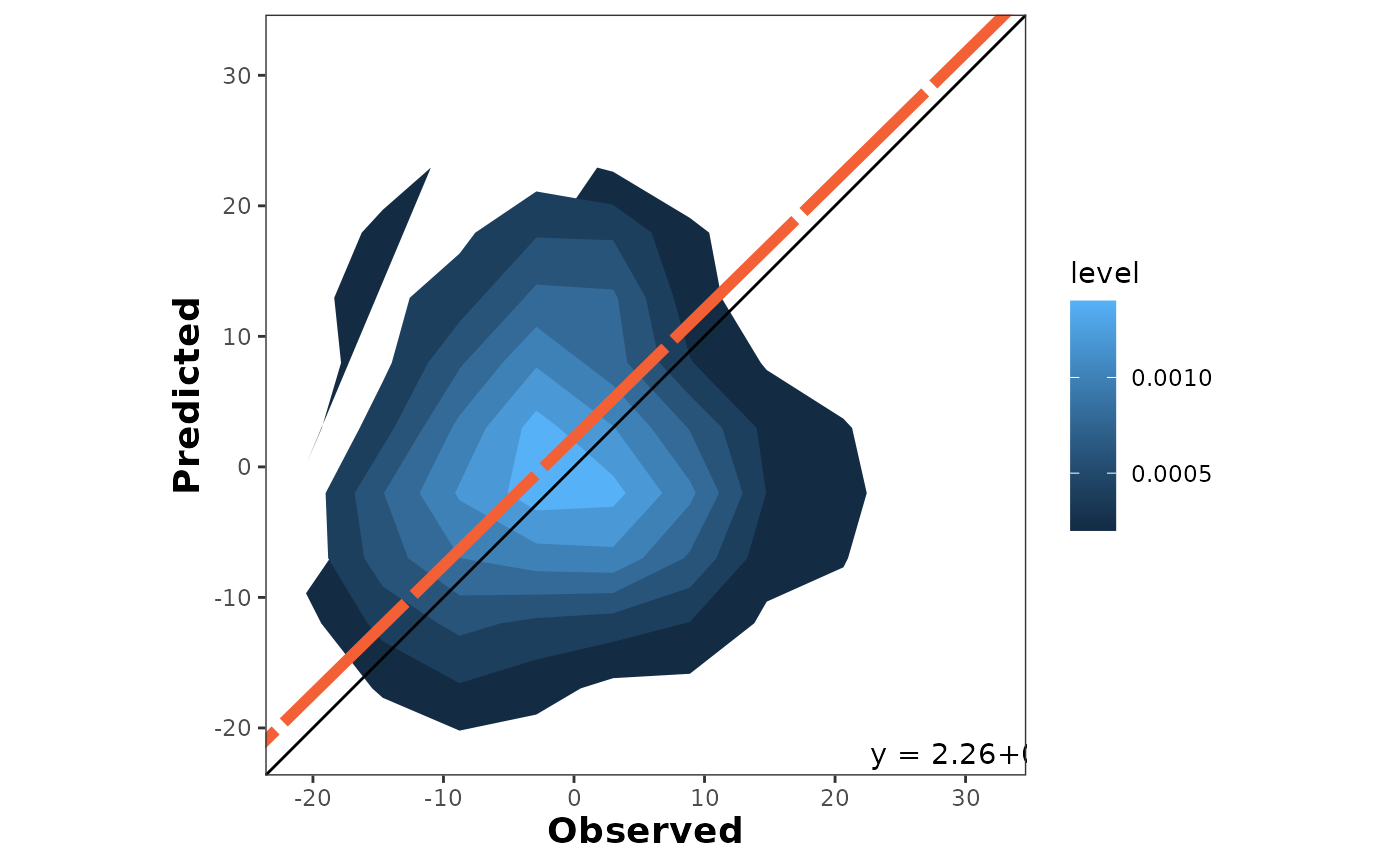It draws a density area plot of predictions and observations with alternative axis orientation (P vs. O; O vs. P).

## Usage

density_plot(
data = NULL,
obs,
pred,
n = 10,
colors = c(low = NULL, high = NULL),
orientation = "PO",
print_metrics = FALSE,
metrics_list = NULL,
position_metrics = c(x = NULL, y = NULL),
print_eq = TRUE,
position_eq = c(x = NULL, y = NULL),
eq_color = NULL,
regline_type = NULL,
regline_size = NULL,
regline_color = NULL,
na.rm = TRUE
)

## Arguments

data

(Optional) argument to call an existing data frame containing the data.

obs

Vector with observed values (numeric).

pred

Vector with predicted values (numeric).

n

Argument of class numeric specifying the number of data points in each group.

colors

Vector or list with two colors '(low, high)' to paint the density gradient.

orientation

Argument of class string specifying the axis orientation, PO for predicted vs observed, and OP for observed vs predicted. Default is orientation = "PO".

print_metrics

boolean TRUE/FALSE to embed metrics in the plot. Default is FALSE.

metrics_list

vector or list of selected metrics to print on the plot.

position_metrics

vector or list with '(x,y)' coordinates to locate the metrics_table into the plot. Default : c(x = min(obs), y = 1.05*max(pred)).

print_eq

boolean TRUE/FALSE to embed metrics in the plot. Default is FALSE.

position_eq

vector or list with '(x,y)' coordinates to locate the SMA equation into the plot. Default : c(x = 0.70 max(x), y = 1.25*min(y)).

eq_color

string indicating the color of the SMA-regression text.

regline_type

string or integer indicating the SMA-regression line-type.

regline_size

number indicating the SMA-regression line size.

regline_color

string indicating the SMA-regression line color.

na.rm

Logic argument to remove rows with missing values (NA). Default is na.rm = TRUE.

## Value

Object of class ggplot.

## Details

It creates a density plot of predicted vs. observed values. The plot also includes the 1:1 line (solid line) and the linear regression line (dashed line). By default, it places the observed on the x-axis and the predicted on the y-axis (orientation = "PO"). This can be inverted by changing the argument orientation = “OP". For more details, see online-documentation

ggplot,geom_point,aes

## Examples

# \donttest{
X <- rnorm(n = 100, mean = 0, sd = 10)
Y <- rnorm(n = 100, mean = 0, sd = 10)
density_plot(obs = X, pred = Y)
#> Warning: The dot-dot notation (..level..) was deprecated in ggplot2 3.4.0.
#> ℹ Please use after_stat(level) instead.
#> ℹ The deprecated feature was likely used in the metrica package.
#>   Please report the issue at